# The MolePage 1

#### WATCH ALL SLIDES

Slide 1The Mole

Slide 2CA Standards

Slide 3## The Mole

1 dozen =

1 gross =

1 ream =

1 mole =

12

144

500

6.02 x 1023

There are exactly 12 grams of carbon-12 in one mole of carbon-12.

Slide 4I didn’t discover it. Its just named after me!

Slide 5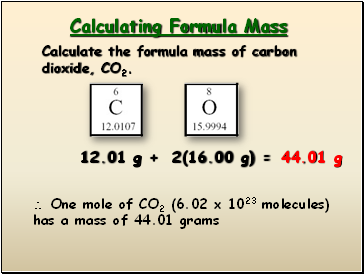## Calculating Formula Mass

Calculate the formula mass of carbon dioxide, CO2.

12.01 g + 2(16.00 g) =

44.01 g

 One mole of CO2 (6.02 x 1023 molecules) has a mass of 44.01 grams

Slide 6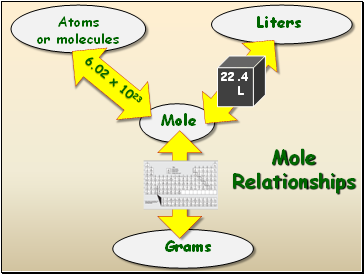Mole Relationships

Mole

Atoms

or molecules

Liters

Grams

6.02 x 1023

Atomic

Mass

22. 4 L

22.4

L

Slide 7## Calculations with Moles: Converting moles to grams

How many grams of lithium are in 3.50 moles of lithium?

3.50 mol Li

= g Li

1 mol Li

6.94 g Li

24.3

Slide 8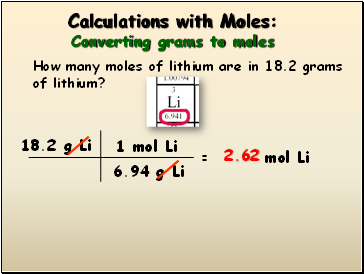## Calculations with Moles: Converting grams to moles

How many moles of lithium are in 18.2 grams of lithium?

18.2 g Li

= mol Li

6.94 g Li

1 mol Li

2.62

Slide 9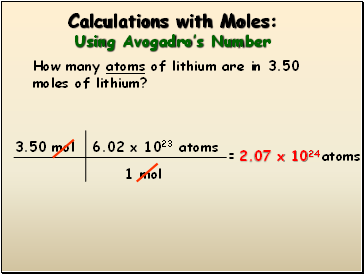## Calculations with Moles: Using Avogadro’s Number

How many atoms of lithium are in 3.50 moles of lithium?

3.50 mol

= atoms

1 mol

6.02 x 1023 atoms

2.07 x 1024

Slide 10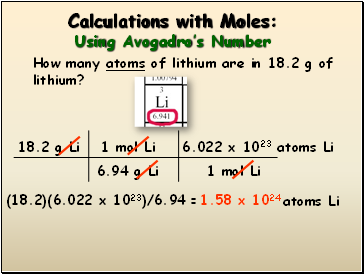Calculations with Moles: Using Avogadro’s Number

How many atoms of lithium are in 18.2 g of

lithium?

18.2 g Li

= atoms Li

1 mol Li

6.022 x 1023 atoms Li

1.58 x 1024

6.94 g Li

1 mol Li

(18.2)(6.022 x 1023)/6.94

Slide 11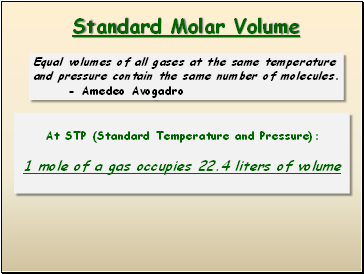## Standard Molar Volume

Equal volumes of all gases at the same temperature and pressure contain the same number of molecules.

At STP (Standard Temperature and Pressure):

1 mole of a gas occupies 22.4 liters of volume

Slide 12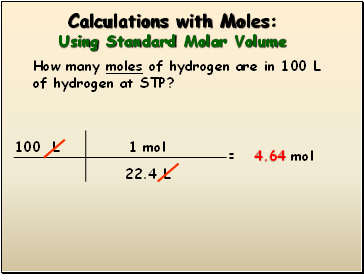Go to page:
1  2